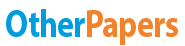# The Efficiency of a Ramp

Essay by   •  October 14, 2017  •  Lab Report  •  1,208 Words (5 Pages)  •  1,301 Views

## Essay Preview: The Efficiency of a Ramp

Report this essay
Page 1 of 5

## The Efficiency of a Ramp

A machine changes the amount of force needed to be applied on an object to make work easier. Some things as simple as a ramp is called a machine because it allows to raise an object from one level to another with less force. However, when using a ramp, friction is encountered due to two objects rubbing against each other causing the machine to lose its efficiency. Some ramps, however, are more efficient than others due to a smaller amount of friction. One factor that effects the efficiency of a ramp is inclination.

This investigation will be conducted to discover the effects different angles have on an efficiency of a ramp.

It is hypothesised that as the angle of the ramp increases, the efficiency of the ramp will also increase. From Fig 1, it can be seen that the two forces operating on an object in an inclined plane is the force due to gravity and the normal force which is perpendicular to the surface of the ramp. As the inclination increases, the normal force will decrease which reduces the overall friction on the object. Since there will be less friction, less energy will be lost to heat with the surface of the ramp which will make it more efficient.

Fig 1: Forces acting on an object in a ramp[pic 1]

Apparatus

• dynamics carts
• ramp
• beam balance
• spring scale calibrated in newtons
• several books
• metre stick
• extra masses
• protractor

Variables

Independent variable: angle of the ramp (causing the height of the ramp to change as well)

Dependent Variable: efficiency of the ramp

Controlled variables: length of the ramp (0.8 m), mass of the cart (0.76 kg), speed of the cart.

Method

1. The mass of the cart was measured.
2. The apparatus was set up as shown in Fig 3.
3. The length of the ramp was measured
4. The height was measured.[pic 2]

Fig 2: Calculating the angle

The angle was calculated using Fig 2 and the following equation:
[pic 3]

1. The force scale was attached to the cart and the average force needed to pull the cart up the ramp at a constant speed was determined.
2. Steps 4, 5 and 6 were repeated four more times using a different slope.[pic 4]

Fig 3: Setting up the apparatus

Results

The following table displays the results obtained after following the method stated above.

 Angle of Ramp (°) Height of Ramp (m) Force (N) Work In (J) Work Out (J) Efficiency (%) Trial 1 Trial 2 Trial 3 Average 3.87 0.054 0.50 0.51 0.64 0.55 0.44 0.40 91 5.02 0.070 0.68 0.71 0.71 0.70 0.56 0.52 93 7.10 0.099 1.0 0.99 0.95 0.98 0.78 0.74 95 8.98 0.125 1.23 1.23 1.23 1.23 0.97 0.93 96 10.6 0.248 1.42 1.45 1.42 1.43 1.13 1.1 97

Fig 4: Results

The Work In was calculated using the formula: [pic 5]

Example: When the angle of the ramp was , the average force was  and the distance over which the force was applied was constant at . [pic 6][pic 7][pic 8]

...

...